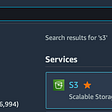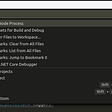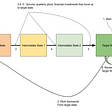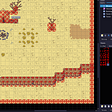# Find maximum sum path by involving elements from two arrays

`class Main {// Function to find the maximum sum path in two given arrays.// The code is similar to the merge routine of the merge sort algorithm public static int findMaxSum(int[] X, int[] Y) {   int sum = 0;   int sum_x = 0, sum_y = 0;   int m = X.length, n = Y.length;   // `i` and `j` denotes the current index of `X` and `Y`, respectively   int i = 0, j = 0;   // loop till `X` and `Y` are empty   while (i < m && j < n) {     // to handle the duplicate elements in `X`   while (i < m-1 && X[i] == X[i+1]) {     sum_x += X[i++];   }// to handle the duplicate elements in `Y`   while (j < n-1 && Y[j] == Y[j+1]) {    sum_y += Y[j++];   }// if the current element of `Y` is less than the current element of `X`   if (Y[j] < X[i]) {     sum_y += Y[j];    j++;   }// if the current element of `X` is less than the current element of `Y`   else if (X[i] < Y[j]) {    sum_x += X[i];    i++;   }   else // if (X[i] == Y[j]) {    // consider the maximum sum and include the current cell's value    sum += Integer.max(sum_x, sum_y) + X[i];   // move both indices by 1 position   i++;   j++;// reset both sums   sum_x = 0;   sum_y = 0;  }}// process the remaining elements of `X` (if any)    while (i < m)     sum_x += X[i++];// process the remaining elements of `Y` (if any)   while (j < n)     sum_y += Y[j++];    sum += Integer.max(sum_x, sum_y);    return sum;  } public static void main(String[] args) {   int[] X = { 3, 6, 7, 8, 10, 12, 15, 18, 100 };   int[] Y = { 1, 2, 3, 5, 7, 9, 10, 11, 15, 16, 18, 25, 50 };   System.out.print("The maximum sum is " + findMaxSum(X, Y)); }}Output:The maximum sum is 199`

--

--

--

## More from Sivareddy Uppathi

Full Stack Software Developer| Programming enthusiast | Loves DS-Algo and System Design

Love podcasts or audiobooks? Learn on the go with our new app.

## How Chaos Engineering plays a vital role in Devops Success## Create S3 Bucket in AWS## You Know More Than We Do## Debug Asp.net Core application running in Docker using vs code## Top #5 .NET Trends that Every Developer should Know## Tactics and Strategy## I have one month to make an MMO: Day 6## Persisting view-state when using a custom backstack: Creating a Flow-like custom backstack (Part 4)## Sivareddy Uppathi

Full Stack Software Developer| Programming enthusiast | Loves DS-Algo and System Design

## 0–1 Knapsack Using Dynamic Programming## Merge Sorted Array🐧